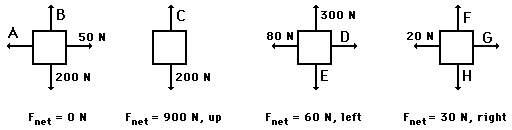Newton's Laws - Lesson 2 - Force and Its Representation

# Determining the Net Force

If you have been reading through Lessons 1 and 2, then Newton's first law of motion ought to be thoroughly understood.

An object at rest tends to stay at rest and an object in motion tends to stay in motion with the same speed and in the same direction unless acted upon by an unbalanced force.

In the statement of Newton's first law, the unbalanced force refers to that force that does not become completely balanced (or canceled) by the other individual forces. If either all the vertical forces (up and down) do not cancel each other and/or all horizontal forces do not cancel each other, then an unbalanced force exists. The existence of an unbalanced force for a given situation can be quickly realized by looking at the free-body diagram for that situation. Free-body diagrams for three situations are shown below. Note that the actual magnitudes of the individual forces are indicated on the diagram.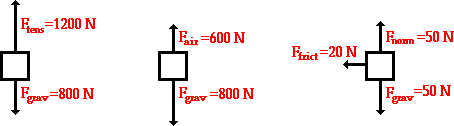In each of the above situations, there is an unbalanced force. It is commonly said that in each situation there is a net force acting upon the object. The net force is the vector sum of all the forces that act upon an object. That is to say, the net force is the sum of all the forces, taking into account the fact that a force is a vector and two forces of equal magnitude and opposite direction will cancel each other out. At this point, the rules for summing vectors (such as force vectors) will be kept relatively simple. Observe the following examples of summing two forces: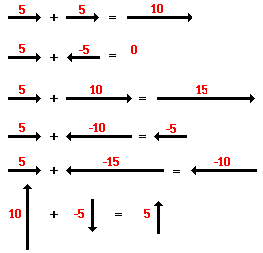Observe in the diagram above that a downward vector will provide a partial or full cancellation of an upward vector. And a leftward vector will provide a partial or full cancellation of a rightward vector. The addition of force vectors can be done in the same manner in order to determine the net force (i.e., the vector sum of all the individual forces). Consider the three situations below in which the net force is determined by summing the individual force vectors that are acting upon the objects.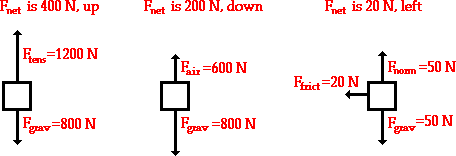### A Net Force Causes an AccelerationAs mentioned earlier, a net force (i.e., an unbalanced force) causes an acceleration. In a previous unit, several means of representing accelerated motion (position-time and velocity-time graphs, ticker tape diagrams, velocity-time data, etc.) were discussed. Combine your understanding of acceleration and the newly acquired knowledge that a net force causes an acceleration to determine whether or not a net force exists in the following situations. Click on the button to view the answers.

 Description of Motion Net Force: Yes or No?See AnswerYes! There is a net force since there is an acceleration.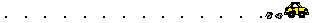See AnswerNo! There is no net force since there is not an acceleration.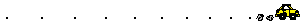See AnswerYes! There is a net force since there is an acceleration.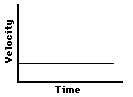See AnswerNo! There is a no net force since there is not an acceleration (zero slope on a v-t graph means zero acceleration).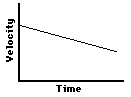See AnswerYes! There is a net force since there is an acceleration (the slope on a v-t graph means acceleration).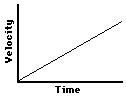See AnswerYes! There is a net force since there is an acceleration (the slope on a v-t graph means acceleration).

### Check Your Understanding

1. Free-body diagrams for four situations are shown below. For each situation, determine the net force acting upon the object. Click the buttons to view the answers.2. Free-body diagrams for four situations are shown below. The net force is known for each situation. However, the magnitudes of a few of the individual forces are not known. Analyze each situation individually and determine the magnitude of the unknown forces. Then click the button to view the answers.